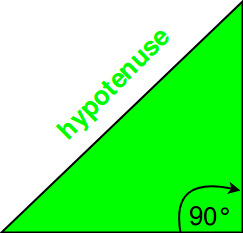# hypot(), hypotf(), hypotl() in C++

The hypot() function in C++ returns the square root of sum of square of arguments passed. It finds the hypotenuse, hypotenuse is the longest side of a right angled triangle. It is calculated by the formula :

` h = sqrt(x2+y2)`

where x and y are the other two sides of the triangle.```Syntax:
double hypot(double x, double y);
float hypot(float x, float y);
long double hypot(long double x, long double y);
```

Examples:

```Input : x=3, y=4
Output :5

Input :x=9, y=10
Output :13.4536
```

Explanation

Parameters : The hypot() takes either 2 or 3 parameters of integral or floating-point type.
Returns :
1. The hypotenuse of a right-angled triangle if two arguments are passed.
2. Distance from the origin to the (x, y, x) if three arguments are passed

Exceptions or Errors
1. hypot(x, y), hypot(y, x), and hypot(x, -y) are equivalent.
2. If one of the arguments is 0, hypot(x, y) is equivalent to fabs called with the non-zero argument
3. If one of the arguments is infinite or undefined, hypot(x, y) returns undefined.

Example Application : Finding the hypotenuse of a right angle triangle given 2 of its other sides.

## Recommended: Please try your approach on {IDE} first, before moving on to the solution.

 `// CPP program to illustrate ` `// hypot() function ` `#include ` `#include ` `using` `namespace` `std; ` `// Driver Program ` `int` `main() ` `{ ` `    ``double` `x = 9, y = 10, res; ` `    ``res = hypot(x, y); ` ` `  `    ``// hypot() returns double in this case ` `    ``cout << res << endl; ` `    ``long` `double` `a, b, result; ` `    ``a = 4.525252; ` `    ``b = 5.767676; ` ` `  `    ``// hypot() returns long double in this case ` `    ``result = hypot(a, b); ` `    ``cout << result; ` `    ``return` `0; ` `} `

Output:

```13.4536
7.33103
```

hypotf() function

hypotf() function is same as the hypot function.The only difference is that the parameter and return type of the function is float type. The ‘f‘ character appended to ‘hypotf‘ stands for float and it signify the parameter type and return type of the function.

```Syntax
float hypotf(float x);
```

C++ program implementation of hypotf()
Here, variables are assigned float type otherwise type mismatch error occurs.

 `// CPP program to illustrate ` `// hypotf() function ` `#include ` `#include ` `using` `namespace` `std; ` `// Driver Program ` `int` `main() ` `{ ` `    ``float` `x = 9.3425, y = 10.0987, res; ` ` `  `    ``// hypotf() takes float values and returns float ` `    ``res = hypotf(x, y); ` `    ``cout << res << endl; ` `    ``return` `0; ` `} `

Output:

```13.7574
```

hypotl() function

hypotl() function is same as the hypot function.The only difference is that the parameter and return type of the function is long double type.The ‘l‘ character appended to ‘hypotl‘ stands for long double and it signify the parameter type and return type of the function.

```Syntax
long double hypotl(long double x);
```

C++ program implementation of hypotl()
Here, variables are assigned long double type otherwise type mismatch error occurs.

 `// CPP program to illustrate ` `// hypotl() function ` `#include ` `#include ` `using` `namespace` `std; ` `// Driver Program ` `int` `main() ` `{ ` `    ``long` `double` `x = 9.3425453435, y = 10.0987456456, res; ` ` `  `    ``// hypotl() takes long double values and returns long double ` `    ``res = hypotl(x, y); ` `    ``cout << res << endl; ` `    ``return` `0; ` `} `

Output:

```13.7575
```

Rated as one of the most sought after skills in the industry, own the basics of coding with our C++ STL Course and master the very concepts by intense problem-solving.

My Personal Notes arrow_drop_upCheck out this Author's contributed articles.

If you like GeeksforGeeks and would like to contribute, you can also write an article using contribute.geeksforgeeks.org or mail your article to contribute@geeksforgeeks.org. See your article appearing on the GeeksforGeeks main page and help other Geeks.

Please Improve this article if you find anything incorrect by clicking on the "Improve Article" button below.

Improved By : Akanksha_Rai

Article Tags :
Practice Tags :

Be the First to upvote.

Please write to us at contribute@geeksforgeeks.org to report any issue with the above content.